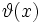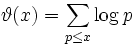# First Chebyshev function

(diff) ← Older revision | Latest revision (diff) | Newer revision → (diff)
Letbe a positive real number. The first Chebyshev function of, denotedor, is defined as:,
where the sum is only over the prime numbers less than or equal to.
• Prime-counting function: Denoted, this simply counts the number of primes less than or equal to.
• Second Chebyshev function: A similar summation, but this time of the von Mangoldt function over all prime powers less than or equal to.